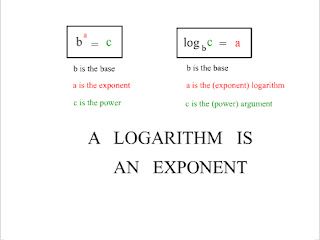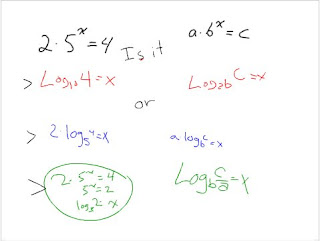## Sunday, April 13, 2008

### Logarithms and Their Laws

Okie please bare with me, I can't write the subscripts so the questions might look funny XD. The first part is somewhat a review, so just scroll down to the 3 laws for the new stuff. Enjoy!

Class started off with a discussion over that flickr project that was due on friday at midnight! But back to math, the first slide was yet another reminder from Mr.K about a logarithm being an exponent. He still insists we're going to forget but we'll prove him wrong riiight?The anatomy of a power is really important. If you know all the terms and what each part is called it'll help when you're solving these log questions..for real.

The first slide were some warm up questions that we've learned. This is a logarithm question so we know what logarithms do right? Say it with me. A logarithm is an exponent! So how I think when I do questions like the ones on the bottom slide is what exponent will give me that power. For example the log2(2), so we know the base is 2 and the power is 1. What to the exponent of 2 will result in a power of 2? That is how we get 1. In math we are always asked to go backwards and forwards soo there isn't an exception in this unit.Rewriting a logarithm to it's exponential expression isn't too hard. First, we take the base and make the exponent in the logarithm it's exponent. An eponential expression turns an exponent to a power so the expression will equal the power. For example we use the question log2(2)=1 and rewrite it to it's exponential expression. The base is 2, the exponent is 1, and the power is 2 so our expression is 2^1=2.To solve the question 2.5^x=4 we turn it into a logarithm. As you can see from the slide above, we had 3 ways the question was solved but only one was correct. The mistake made was multiplying 2 and 5. You can't arbitrarely multiply the numbers. The correct way to do it was the 3rd choice. What must be done first is to divide everything by 2 so the 5^x is by itself. Then we turn it into a logarithm log5 (2) = x. If the question asks for the exact value of x that is how you would write it since log5 (2) is not a whole number.

On Friday's lesson we also learned how to write our answers in an elegant way again. For example we'll use the question y =Loga 3x and solve for x. Once again we write it in its exponential expression. 3x = a^y then isolate x. To make our work elegant we would write the answer as (1/3)(a^y) instead of (a^y/3).On the slide above, we graphed one of the logarithms. First we shall ignore the absolute value signs and save it for later. Then we turn y = log4 x into its exponential expression and we get 4^y=x. After this step we use our past knowledge of inverses, which we get by switching x and y. So the inverse of 4^y =x is 4^x=y and we know how to graph this now. Unfortunately that isn't the graph we want. So after graphing 4^x=y we take its x and y points and switch em to get the graph we do want! Now it is time to deal with those absolute value signs. We learned in the past that whenever we have an absolute value to just take the negative values and flip them up. You may be wondering what the word cusp up on the slide is. A cusp is different from a corner. It is a point where 2 points meet and makes a very sharp curve. As for where it is increasing the answer is (0, infinite) because that's where the x values start to increase. (0,1) is where the x values start to decrease from what we see on the graph. Notice that we did not put square brackets around the 0 on both because it cannot be increasing and decreasing at the same time. A helpful fact we learned on Friday was if a questions asks "where" it wants x values. If it asks what it is for the y values.

Now it is time for what I thought was the most important part of the lesson, the laws! Let's start with the product law. It is the same concept as (3^5)(3^7)=3^12. When we have powers with the same base we are able to add the exponent. So we can use that same rule for the question log2 (8.16). Since it is multiplying 2 powers with the same base, we know that it must be adding exponents (logs are exponents remember). So the question should look like this now: log2 8 + log2 16. The log2 8 = 3 and the log2 16 = 4. Those are the exponents and we add them to get 7. What if we get a question like express loga 2 + loga 3 as a single log? It is exactly what we did up there but going backwards this time. They both have the same base of a and when we add that means the the powers (2 and 3) are being multiplied. The work should look like this: loga (2.3) and can be further simplified to be loga 6.

Another law we learned was the quotient law. It is also the same concept as (3^7)/(3^5). When we are dividing the same bases we can subtract the exponents. Again it's the same concept when we do a question like log2 (32/128). Since it is dividing the powers it must mean it is subtracting exponents. So we can write this question like this now: log2 32 - log2 128 because logs are exponents. Guess what we'll do next?! If you guessed work backwards...then you're right. We'll use the question loga 5 - loga 4. In this question it's subtracting logs, which is like subtracting 2 exponents. When are we able to subtract exponents? Only when we are dividing powers with the same base. So with that we can write loga 5 - loga 4 as loga (5/4) and that's the answer.

Finally, the last law, which is the power law. Just like the two other laws, it has the same concept as saying (5^3)^7. When there is a power to the exponent of something we can multiply the exponents. So for that question it'll be (5^21). Now using that concept, we can apply it to a question like log2 8^5. Since the power, which is 8 is to the exponent of 5, we know that it must be multiply exponents. Just like multiplying 3 by 7 we multiply the log2 8 by 5 because log is an exponent. We can write the question like this now: 5(log2 8) which is 15. Once again we'll do this process backwards. Let's simplify 3loga 2. The answer is loga 2^3. This is because the exponent, which is the log is being multiplied by a number. Since we are multiply, we know that it must be multiplying the power with an exponent.

That was a really really long scribe post. It was really hard wording the power law. But the concept of a log being an exponent is really helpful if you understand that part. Yes, this is long enough. Too bad, I didn't even get to talk about Star Trek.

Duck duck duck...goose! *points at kristina* You're next scribe.

#### 1 comment:

m@rk said...

Joyce,

I must say that it was a really long scribe post, but it was very well done. Like i said on Kristina's scribe post, I like how you inserted an image so that your readers can easily link to what you are saying. It saves them lots of time and most of all makes them learn visually. I like to consider myself as a visual learner so this post really helped me relearn all this laws.

-m@rk